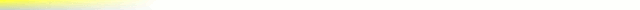# [mkgmap-dev] DP Filter and areas at Resolution=24

From Johann Gail johann.gail at gmx.de on Thu Nov 26 23:38:05 GMT 2009

```> One idea for rounding at that level:
>
> Most annoying is that rectangles that are buildings aren't rectangles anymore after rounding. As the OSM data in itself probably isn't accurate at the 2 m level perhaps we could do "tweak" the coordinates so that rectangles stay rectangles (I know, the projection has an influence as well)? This would make the maps much better looking.
>
>
>
For lines the rounding is simply done by setting the coord to the
nearest garmin unit by distance.
For polygons (and maybe even for lines) not the nearest garmin unit
should be choosen, but the garmin unit, which has the smallest changes
to the angle. So the least influence to the outline should be achieved.

An possible algorithm could be:

foreach point in the polygon do:
calculate the real angle (with unrounded coords!)
round coordinates to the upper left garmin unit, calculate angle a1
round coordinates to the lower left garmin unit, calculate angle a2
round coordinates to the upper right garmin unit, calculate angle a3
round coordinates to the lower right garmin unit, calculate angle a4
choose the coordinate with the least difference to the real angle.

```